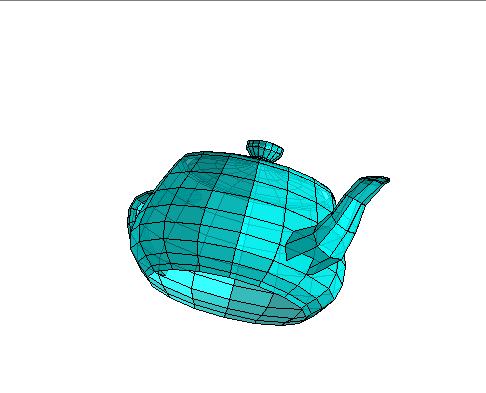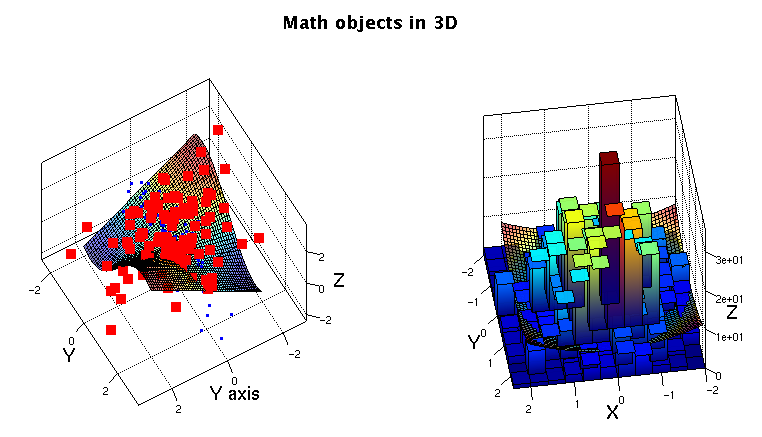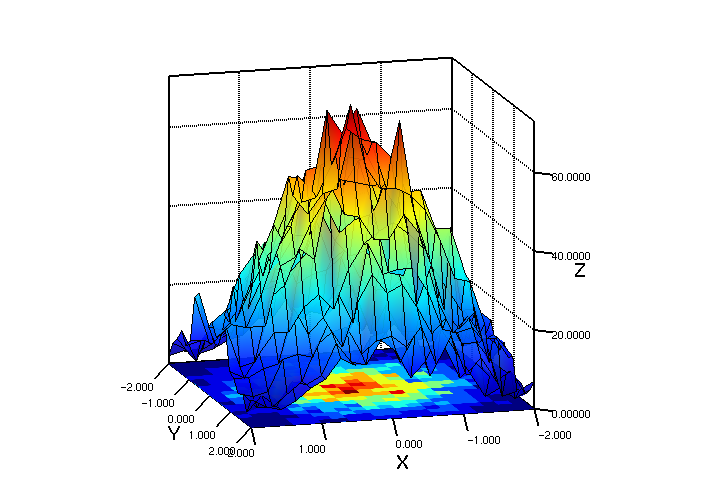# DMelt:Plots/5 Plots in 3D

Limitted access. First login to DataMelt if you are a full DataMelt member. Then login to HandWiki as a user.

# Plots in 3D

DMelt contains several powerful engines to draw functions, histograms, data and various primitives in 3D. Graphics is fully interactive thus one can rotate objects, insert text etc. Many of such engines are 100% native to Java.

Several Java canvases which can be used to show math objects are shown below:

Canvas Descriptionjhplot.HPlot3D is used for plotting data in 3Djhplot.H2D,jhplot.P2D,jhplot.P3D,jhplot.F2Djhplot.HPlotXYZ is used for plotting data and geometrical objects in 3D using OpenGL. Can be used to showjhplot.H2D,jhplot.P3D,jhplot.F2Djhplot.HPlot3DP is used for plotting parametric and non-parametric functions such asjhplot.FPRjhplot.HVisAd to show data in 2D/3D for visualization and analysis of numerical data usingVisAdjhplot.HPlotMX as before, for plotting parametric and non-parametric functions for mathematical "exhibits"jhplot.HJavaView 3D geometry viewer and a mathematical visualization software from http://www.javaview.de

# Showing data in 3D (X-Y-Z plots)

There are several pads to show data in 3D:jhplot.HPlot3D (histograms, functions, data points) andjhplot.HPlot3DP (used for parametric functions). All such canvases are 100% JAVA libraries.

DMelt has many engines to render 3D graphics in fully interactive way. This is rather large topic. In this section we just show a few basic examples.

# 3D graphics from OFF files

Here is an example to read OFF files. In this case we read the file "teapot.off"

from jhpro.engine3d import *
view=HEngine3D("teapot.off")
view.setBounds(10, 10, 600, 400)
view.setVisible(1)Read about the definitions of the OFF file Section DMelt:Plots/OFF_Files. This engine does not support showing mathematical functions nor displaying axes. There are several methods to change the color, transparency, and the solid style.

This is the most powerful engine to render 3D graphics and mixing mathematical objects and graphical primitives.

## Scientific plots with OpenGL

DMelt includesjhplot.HPlotXYZ class which relies on on JOGL2. You can easily deploy native OpenGL engine for fast rendering of plots. This is done via the JZY3D library . This library allows the generation of pictures without opening a frame, as well as multiple views of the same scene in several panels.

Here is an example which shows how to draw interactive 3D objects in the 3D canvas using Java and Jython. The code shown below is written in Jython/Python and contains only 10 lines.

Use the method "export("image.png")" to save the image.

## Functions in 3D

One can plot functions in 3D, overlaying any number of functions and applying custom colors. In many cases, DMelt relies on on JOGL2 via the JZY3D library . Below a simple Jython example which shows this using 10 lines of code (similarly, one can rewrite this example in Java):

Now we will make a more complicated function: instead of giving a string defining a function, we will build a complex function using the Python language. Our function will look as this:

if x>0:   z=a*y*x+b*sin(y)
if x<0:   z=c*cos(x*y)+sin(y)

As you can see, it has 3 parameters a,b,c. Now let us create a small code snipped to draw such function. We put comments which explain each step:

Another way to draw functions in3D is to usejhplot.HJavaView canvas. You can plot the standardjhplot.F2D functions usingjhplot.HJavaView. You can use either the "draw(F2D)" method, or "add(F2D)" method. The latter returnsjv.project.PgGeometry object which can be used to modify style of the function (color, transparency). For example,

from jhplot import HJavaView,F2D
c1=HJavaView()
f1=F2D("x*y",-1,1,-1,1)
c1.draw(obj)

Similarly, one can draw arrays of functions, or one can repeat "draw()" to overlay functions.

## Math objects in 3D

One can draw the following mathematical objects: arrays of points with custom sizes and color, histograms and mathematical functions in 3D. All such objects can be overplayed on the same canvas. Below is a small script which plots several datasets in 3D (with blue and red points), functions as surfaces and H2D histograms:

The output of this code is:Histograms can be shown using different style. In the above example we use "rainbow" color style for the histogram. One can also show it using solid color or as a surface:

The output of this code isGenerally, there is significant difference between the 2 main canvases for 3D mathematical visualization.jhplot.HPlot3D does not use hardware acceleration using native OpenGL libraries, On the contrary,jhplot.HPlotXYZ class relies on Jzy3d library with JOGL, you can easily deploy native OpenGL.jhplot.HPlotXYZ is faster for interactive plotting of many mathematical objects (functions, data points, histograms).

Data and mathematical objects in 3D can be shown usingjhplot.HVisAd canvas that builds a frame integrated with the VisAd, a Java component library for interactive and collaborative visualization and analysis of numerical data. It provides a general display that supports interactive 3D viewer, multiple data views, direct manipulation. The display model has been adapted to Java3D.

There is dedicated VisAd section describing this topic.

## Showing 3D math exhibits

Mathematical functions (parametric and non-parametric) can be shown usingjhplot.HPlotMX Java class.

Here is a simple Jython script which creates a predefined paraboloid:

from jhplot import *
c1=HPlotMX("vmm3d.surface.parametric.Paraboloid")
c1.visible()

Here we simply call the class name that defines "Paraboloid". Please read the section DMelt:Plots/6_Math_Exhibits for more detail.